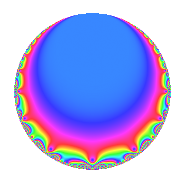# Properties

 Label 4005.2.a.bLevel 4005 Weight 2 Character orbit 4005.a Self dual Yes Analytic conductor 31.980 Analytic rank 1 Dimension 1 CM No Inner twists 1

# Related objects

## Newspace parameters

 Level: $$N$$ = $$4005 = 3^{2} \cdot 5 \cdot 89$$ Weight: $$k$$ = $$2$$ Character orbit: $$[\chi]$$ = 4005.a (trivial)

## Newform invariants

 Self dual: Yes Analytic conductor: $$31.9800860095$$ Analytic rank: $$1$$ Dimension: $$1$$ Coefficient field: $$\mathbb{Q}$$ Coefficient ring: $$\mathbb{Z}$$ Coefficient ring index: $$1$$ Fricke sign: $$1$$ Sato-Tate group: $\mathrm{SU}(2)$

## $q$-expansion

 $$f(q)$$ $$=$$ $$q - 2q^{4} - q^{5} - 4q^{7} + O(q^{10})$$ $$q - 2q^{4} - q^{5} - 4q^{7} - 2q^{11} + 4q^{13} + 4q^{16} + 6q^{17} + 2q^{20} - 5q^{23} + q^{25} + 8q^{28} + 5q^{29} - 2q^{31} + 4q^{35} - 2q^{37} - 5q^{41} + 2q^{43} + 4q^{44} + 8q^{47} + 9q^{49} - 8q^{52} - 8q^{53} + 2q^{55} + 9q^{59} + 14q^{61} - 8q^{64} - 4q^{65} + 15q^{67} - 12q^{68} - 12q^{71} - 15q^{73} + 8q^{77} - 5q^{79} - 4q^{80} + 11q^{83} - 6q^{85} - q^{89} - 16q^{91} + 10q^{92} - 5q^{97} + O(q^{100})$$

## Embeddings

For each embedding $$\iota_m$$ of the coefficient field, the values $$\iota_m(a_n)$$ are shown below.

For more information on an embedded modular form you can click on its label.

Label $$\iota_m(\nu)$$ $$a_{2}$$ $$a_{3}$$ $$a_{4}$$ $$a_{5}$$ $$a_{6}$$ $$a_{7}$$ $$a_{8}$$ $$a_{9}$$ $$a_{10}$$
1.1
 0
0 0 −2.00000 −1.00000 0 −4.00000 0 0 0
 $$n$$: e.g. 2-40 or 990-1000 Significant digits: Format: Complex embeddings Normalized embeddings Satake parameters Satake angles

## Inner twists

This newform does not admit any (nontrivial) inner twists.

## Atkin-Lehner signs

$$p$$ Sign
$$3$$ $$1$$
$$5$$ $$1$$
$$89$$ $$1$$

## Hecke kernels

This newform can be constructed as the intersection of the kernels of the following linear operators acting on $$S_{2}^{\mathrm{new}}(\Gamma_0(4005))$$:

 $$T_{2}$$ $$T_{7} + 4$$ $$T_{11} + 2$$